﻿ 2, 3, 4 Wire RTD PT100 Temperature Sensor a Plurality of Different Connection Method_

China sensor manufacturers

# 2, 3, 4 Wire RTD PT100 Temperature Sensor a Plurality of Different Connection Method

Temperature is a very important physical quantity in work control. Sensors used for temperature measurement mainly include thermal resistance, thermistor, thermocouple, semiconductor temperature sensor and so on. These sensors have their own characteristics and application areas, so designers must choose the appropriate sensors according to their specific applications.2, 3, 4-wire PT100 temperature sensor

Thermal resistance (RTD) is a temperature sensor with a high precision that is wound on a support using a thin wire and has a resistance value varying from temperature. The operating temperature range is -200 ° C to + 850 ° C. Common RTD materials are nickel, copper, platinum, etc., among which 100 ohm (0 ° C) platinum thermal resistance is the most common. Due to the very stable physical and chemical properties of platinum metal, platinum resistance is widely used due to its high precision, long-term stability, good repeatability and fast response.
The following formula describes the temperature characteristics of Pt100. From the formula, it can be seen that the temperature and resistance characteristics of Pt100 are obviously nonlinear. However, compared with thermocouples and thermistors, the linearity of Pt100 is much better, so nonlinear correction is easier. Early nonlinear corrections were mostly implemented by complex analog circuits. With the development of electronic technology, in practical applications, the single-chip microcomputer is generally used for table-checking and interpolation to nonlinearly correct Pt100.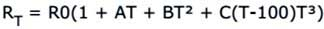Because thermal resistor is a passive device, it needs external excitation to work in the process of using. In the process of using, and it is necessary to pay attention to the magnitude of the excitation current during use. Excessive current causes the Pt100 to heat up itself, resulting in measurement errors. Therefore, the signal conditioning circuit must be carefully designed according to the measurement requirements during the design process to ensure that the error caused by the self-heating of the sensor is within an acceptable range.

One, Why use 3-wire system and four-wire system
Because the temperature coefficient of Pt100 is only 0.385Ω/°C. If the distance between the sensor and the controller is long and a long lead is required, the measurement error due to the lead resistance may be severe. For example, suppose a Pt100 is connected to the controller via a 100-foot (30-meter) copper lead. The resistance of the 30-gauge copper wire is 0.105 Ω/ft. If the conventional two-lead connection method is used, as shown in the figure below, a total of 21Ω resistance is generated on the lead, which is equivalent to the measurement error of 55%!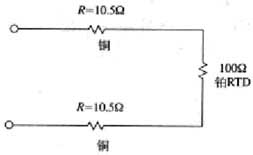In order to solve this problem, in addition to the two-wire connection method, the Pt100 has three-wire and four-wire connection methods to reduce or even eliminate the measurement error caused by the lead resistance.

two, 2-, 3-, 4-wire connection method for constant current source excitation
As mentioned above, the Pt100 is a resistive temperature sensor whose nature of temperature measurement is actually measuring the resistance of the sensor. Usually, the resistance is converted into voltage or current signals for measurement, and the measurement result is linearized by the single chip microcomputer. There are two options for temperature measurement in the general Pt100:
1. A constant current source is designed to calculate the temperature by measuring the voltage on Pt100 through Pt100 thermal resistance.
2. With a Wheatstone bridge, three of the four resistors of the bridge are constant and the other uses a Pt100 thermal resistor. When the resistance value of Pt100 changes, the test end will generate an electric heating difference, and thus the electric heating difference is used to calculate the temperature.
There is also a class of measurement methods called “proportional method”, which can be seen as an improvement of the first type of measurement method, which will be described in detail later.

The connection method of the two-wire constant current source excitation is shown in the figure below. The constant current source is output through Pt100, and the voltage across Pt100 is measured to be converted into temperature. As mentioned earlier, this connection causes a large error when the Pt100 leads are long. Therefore, the more practical ones are the three-wire system and the four-wire system.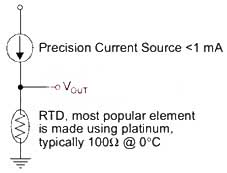The four-wire wiring method (Kelvin connection method) is shown below. The excitation and measurement ends of the Pt100 are respectively taken out by two different sets of leads. Since the voltage measurement point is at the lead connection of the Pt100, the lead excitation voltage does not affect the measurement result.
The four-wire wiring method is widely used in laboratory temperature measurement with its high precision. However, due to factors such as cost and wiring convenience, the three-wire system is more widely used in industrial control sites.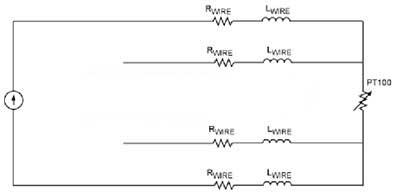The following figure shows a typical wiring method for a three-wire Pt100 excited by a constant current source. The basic principle is to assume that the three leads of the Pt100 use the same type of cable of the same length and have the same resistance. That is, Rw1 = Rw2 = Rw3 in the figure below, using the measuring end (Rw2 lead) to compensate the lead resistance.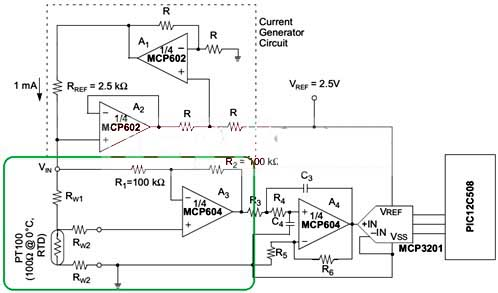Specifically, for the wiring method in the figure, the operation amplifier A3 in-phase input voltage V+=Vpt100+VRw3, and the operation amplifier A3 reverse input voltage V-=VRw1+Vpt100+VRw3. Based on the assumption of Rw1=Rw2=Rw3, there is Vrw1 = Vrw3. The output of op amp A3 is Vo = 2V+ - V- = Vpt100. That is, the influence of the lead resistance on the measurement result is theoretically eliminated.

Third, the 2- 3-wire connection method of Wheatstone Bridge
The Wheatstone Bridge is another common connection to the Pt100. The two-wire connection method is shown in the figure below. Obviously, when the Pt100 lead is long, a large error is introduced.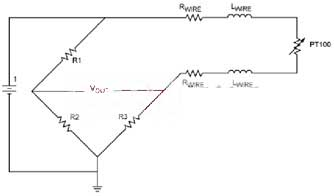The 3-wire Pt100 Wheatstone bridging method is shown in the figure below. The Wheatstone bridge output formula is as follows. If R3 = Rpt100 is selected, the measurement error introduced by the lead resistance can be theoretically eliminated.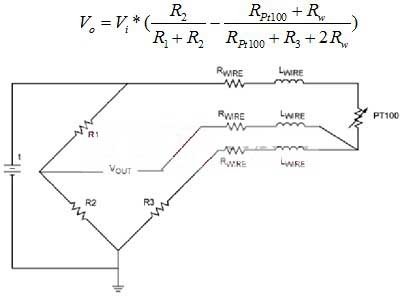Four. 2-, 3-,4-wire system wiring method in Pt100 proportional method measurement
The essence of the proportional method is to connect Pt100 in series with a reference resistor and apply an excitation source. Since the current flowing through Pt100 is the same as the current of the reference resistor, the voltage ratio of the two is the resistance ratio. The voltage across the reference resistor is used as the AD converter reference voltage to AD convert the voltage across the Pt100. Then, in the formula, D is the ADC conversion result, and N is the ADC number of bits.Since the current flowing through Pt100 is the same as the current of the reference resistor, this circuit has a lower requirement on the excitation source than the above two structures. Due to its simple structure, few precision components and high measurement accuracy, the proportional measurement circuit has been recognized by more and more people.

The figure below shows the two-wire Pt100 connection method of the proportional method. Obviously, when the Pt100 lead is long, a large error will be introduced.

In this circuit, the two-wire Pt100 can be directly replaced with the four-wire Pt100, and the four-wire connection method can be changed to eliminate the lead error.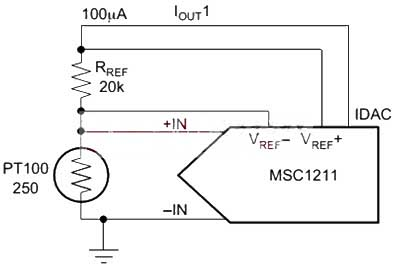The figure below shows the proportional method 3-wire Pt100 connection method, which uses two constant current sources with equal output to compensate the lead resistance.﻿
﻿